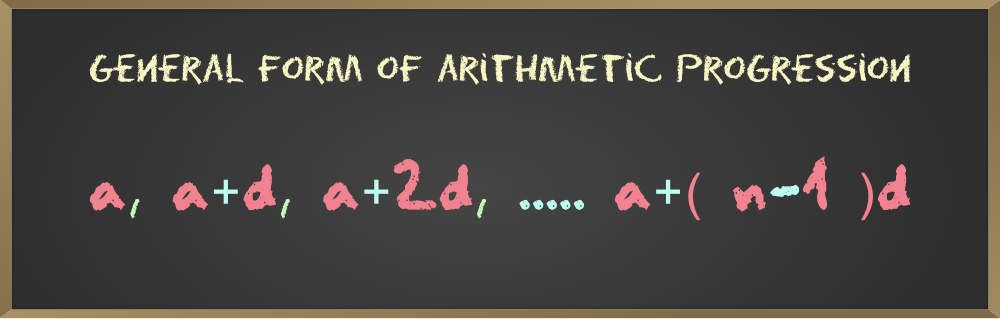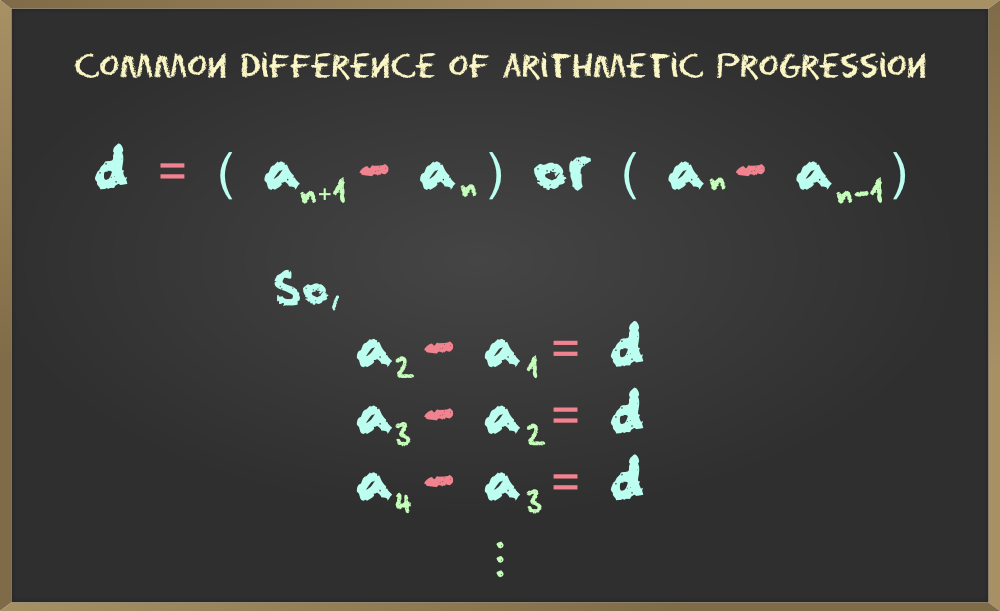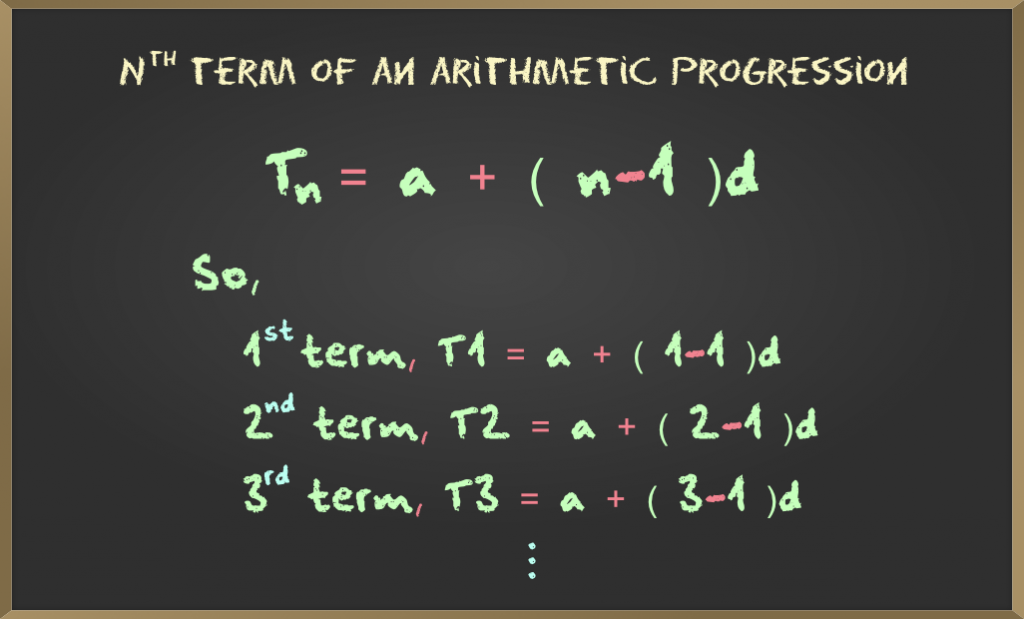Open in App
Not now

# Arithmetic Progression – Common difference and Nth term | Class 10 Maths

• Last Updated : 27 Oct, 2020

Arithmetic Progression is a sequence of numbers where the difference between any two successive numbers is constant. For example
1, 3, 5, 7, 9……. is in a series which has a common difference (3 – 1) between two successive terms is equal to 2. If we take natural numbers as an example of series 1, 2, 3, 4… then the common difference (2 – 1) between the two successive terms is equal to 1.

In other words, arithmetic progression can be defined as “A mathematical sequence in which the difference between two consecutive terms is always a constant“.

We come across the different words like sequence, series, and progression in AP, now let us see what does each word define –

• Sequence is a finite or infinite list of numbers that follows a certain pattern. For example 0, 1, 2, 3, 4, 5… is the sequence, which is infinite sequence of whole numbers.
•

• Series is the sum of the elements in which the sequence is corresponding For example 1 + 2 + 3 + 4 + 5….is the series of natural numbers. Each number in a sequence or a series is called a term. Here 1 is a term, 2  is a term, 3 is a term …….
•

• Progression is a sequence in which the general term can be expressed using a mathematical formula or the Sequence which uses a mathematical formula that can be defined as the progression.## What is the Common difference of an AP?

The common difference in the arithmetic progression is denoted by d. The difference between the successive term and its preceding term. It is always constant or the same for arithmetic progression. In other words, we can say that, in a given sequence if the common difference is constant or the same then we can say that the given sequence is in Arithmetic Progression.

• The formula to find common difference is d = (an + 1 – an ) or d = (an – an-1).
• If the common difference is positive, then AP increases. For Example 4, 8, 12, 16….. in these series, AP increases
• If the common difference is negative then AP decreases. For Example -4, -6, -8……., here  AP decreases.
• If the common difference is zero then AP will be constant. For Example 1, 2, 3, 4, 5………, here AP is constant.

The sequence of Arithmetic Progression will be like a1, a2, a3, a4,…Example 1: 0, 5, 10, 15, 20…..

```here,
a1 = 0, a2 = 5, so a2 - a1 = d = 5 - 0 = 5.
a3 = 10, a2 = 5, so a3 - a2 = 10 - 5 = 5.
a4 = 15, a3 = 10, so a4 - a3 = 15 - 10 =5.
a5 =20, a4 =15, so a5 -a4 = 20 - 15 = 5.

```

From the above example, we can say that the common difference is “5”.

Example 2: 0, 7, 14, 21, 28…….

```here,
a1 = 0, a2 = 7, so a2 - a1 = 7 - 0 = 7
a3 = 14, a2 = 7, so a3 - a2 = 14 - 7 = 7
a4 = 21, a3 = 14, so a4 - a3 = 21 - 14 = 7
a5 =28, a4 = 21, so a5 -a4 =28 - 21 = 7

```

From the above example, we can say that the common difference is “7”.

## How to Find the Middle term of an AP?

To find the middle term of an arithmetic progression we need the total number of terms in a  sequence. We have two cases:

Even: If the number of terms in the sequence is even then we will be having two middle terms i.e (n/2) and  (n/2 + 1).n

Odd: If the number of terms in the sequence is odd then we will be having only one middle terms i.e (n/2).

Example 1:

```If n = 9 then,
Middle term = n/2 = 9/2 = 4.
```

Example 2:

```If n = 16 then,
First middle term = n/2 = 16/2 = 8.
Second middle term = (n/2) + 1 = (16/2) + 1 = 8 + 1 = 9.

```

## What is the Nth term of an AP?

To find the nth term of an arithmetic progression, We know that the A.P series is in the form of a, a + d, a + 2d, a + 3d, a + 4d……….

The nth  term is denoted by  Tn. Thus to find the nth term of an A.P series will be :Example: Find the 9th term of the given A.P sequence: 3, 6, 9, 12, 15………..?

Step 1: Write the given series.

```Given series = 3, 6, 9, 12, 15...........

```

Step 2: Now write down the value of a  and n from the given series.

```a = 3, n = 9

```

Step 3: Find the common difference d by using the formula  (an+1  – an).

```d = a2 - a1 ,
here  a2 = 6 and a1 = 3
so d = (6 - 3) = 3.

```

Step 4: We need to substitute values of a, d, n  in the formula (Tn = a + (n – 1)d).

```Tn =  a + (n - 1)d
given n = 9.

T9 = 3 + (9 - 1)3

= 3 + (8)3

= 3 + 24 = 27

```

Therefore the 9th  term of given A.P series  3, 6, 9, 12, 15………. is “27”.

My Personal Notes arrow_drop_up